Question Pool Polynomials

# Polynomials - SAMAGRA Question Pool & Answers | Class 10 English Medium

Kerala Syllabus SAMAGRA SCERT SAMAGRA Question Pool for Class 10 English Medium Maths PolynomialsQn 1.

The  area of a rectangle is represented by the polynomial P(x) =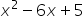,

a) If the length is (x - 1), find the breadth as a first degree polynomial

b) If the length is 5 what is its breadth?

Get Free Study Materials + 1 Week Free Trial of BrainsPrep Class 10 English Medium Tuition

Qn 2.

P(x) is a second degree polynomial.

P(2)=0, and P(-1)=0 then,

a) Which of the following is a factor of P(x)?

i) (x + 2) ii) (x + 1) iii) (x + 3) iv) (x – 1)

b) Find the solutions of the equation p(x) = 0

a) (x + 1)

Get Free Study Materials + 1 Week Free Trial of BrainsPrep Class 10 English Medium Tuition

Qn 3.

If P(x)=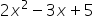, then

a) Find P(0)

b) Check whether (x – 1) is a factor of this polynomial

Get Free Study Materials + 1 Week Free Trial of BrainsPrep Class 10 English Medium Tuition

Qn 4.

If P(x) = (x – 1) (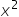– x – 6)

i) Find P(1)

ii) Write P(x) as the product of three first degree polynomials.

Get Free Study Materials + 1 Week Free Trial of BrainsPrep Class 10 English Medium Tuition

Qn 5.

In the given polynomial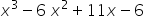a) Find P(1)

b) Write one factor of the polynomial

Get Free Study Materials + 1 Week Free Trial of BrainsPrep Class 10 English Medium Tuition

Qn 6.

(x-3)(x-2) =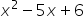, then find the solution of the equation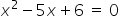Get Free Study Materials + 1 Week Free Trial of BrainsPrep Class 10 English Medium Tuition

Qn 7.

The polynomial P(a) = a2+50a represents the surface area of a square pyramid with base edge 'a', then

a) What is the slant height of this pyramid ?

b) Find the total surface area of the pyramid with base edge 10 units.

a) 2al = 50

Get Free Study Materials + 1 Week Free Trial of BrainsPrep Class 10 English Medium Tuition

Qn 8.

Consider  the polynomial P(x) = x2-kx-7,

a) if P(1) = 3 , Find the value of k.

b) Write a second degree polynomial with factor  (x - 1)

a) 12-k1-7 =

Get Free Study Materials + 1 Week Free Trial of BrainsPrep Class 10 English Medium Tuition

Qn 9.

P(x) is a second degree polynomial. One factor of P(x) is (x – 1)

and if P(7)=0 then

a) Write P(x) as the product of two first degree polynomials.

b) Find the values of x for which P(x) = 0

P(x) =

Get Free Study Materials + 1 Week Free Trial of BrainsPrep Class 10 English Medium Tuition

Qn 10.

If x-1, x+1 are the factors of ax3+bx2+cx+d then

i) Prove that   a=-c,  b=-d

ii) If x-1 and x+1 are factors of ax3+bx2-5x+3, what is a and b?

x – 1 is a factor.

P(1) =

Get Free Study Materials + 1 Week Free Trial of BrainsPrep Class 10 English Medium Tuition

Qn 11.

In the polynomial P(x) = 2x3-x2-8x+4

a) Find P(0).

b) Find  p (1/2)

c) Write one factor of P(x).

a) P(0) = 203-02-80+4=

Get Free Study Materials + 1 Week Free Trial of BrainsPrep Class 10 English Medium Tuition

Qn 12.

a) Manu is asked to write the polynomial x2+7x+6  as the product of first degree polynomials. He wrote as follows and stopped

x2+7x+6 = (x-a)(x-b)

x2+7x+6 = x2 - (a+b)x + ab

Help Manu to solve the problem.

b) Find the solutions of the equation

x2+7x+6=0

x2+7x+6 = (x-a)(x-b)

x2+7x+6 =x2- (a+b)x + ab

For identifying a+b = -7, and

Get Free Study Materials + 1 Week Free Trial of BrainsPrep Class 10 English Medium Tuition

Qn 13.

p(x) = ax2 + bx + 5

a) Find p(1)

b) (x-1) is a factor of p(x). Prove that a+b-5 = 0

c) If (x+1) is a factor of p(x),then find the relation between a and b?

d) If(x2 -1) is a factor of p(x), then find the value of  a and b?

p(x) = ax2 + bx + 5

a. p(1) = a + b + 5

Get Free Study Materials + 1 Week Free Trial of BrainsPrep Class 10 English Medium Tuition

Qn 14.

p(x) = (x +2) (x – 3) + k, (x-2) is a factor of p(x)

a.Find  k

b. p(x) Examine whether (x + 1) is a factor of p(x)

c. Which number should be added to p(x) to make (x +3) a factor of it?

p(2) = (2 + 2) (2 – 3) + k = 0

k = 4

Get Free Study Materials + 1 Week Free Trial of BrainsPrep Class 10 English Medium Tuition

Qn 15.

a)p(x) =3x2 + 16x +5 . Write p(x) as a product of two first degree polynomials.

b) Can we write 2x2 + 5x + 6 as the product of two first degree polynomials?

Get Free Study Materials + 1 Week Free Trial of BrainsPrep Class 10 English Medium Tuition

Qn 16.

p(x) = 2x2 – x – 6 ; 2 is a solution of  p(x) =0

a) Write a factor of p(x)

b) Write p(x) a product of two first degree polynomials.

a) (x –

Get Free Study Materials + 1 Week Free Trial of BrainsPrep Class 10 English Medium Tuition

Qn 17.
Write a polynomial p(x) such that p(1) = 0, p(2) = 0, p(3) = 0.

x – 1, x – 2, x – 3 are factors of p(x)

Get Free Study Materials + 1 Week Free Trial of BrainsPrep Class 10 English Medium Tuition

Qn 18.

p(x) = x3 – 2x2 – 5x + k. (x + 2) is a factor of p(x). Find the value of k?

P(-2) = 0

(-2)3 – 2(-2)2 – 5(-2) + k =

Get Free Study Materials + 1 Week Free Trial of BrainsPrep Class 10 English Medium Tuition

Qn 19.

p(x) =x2 – x – 15 . Which number is added to p(x) to make (x – 5) a factor of it.

Ans p(x) = x2 – x – 15

If(x – 5) is a factor of  p(x) then p(5) = 0

p(5) = 52 – 5 – 15 =

Get Free Study Materials + 1 Week Free Trial of BrainsPrep Class 10 English Medium Tuition

Qn 20.

Write p(x) = x2 – 4x – 21 as the product of two first degree polynomials.

p(x) = x2 – 4x – 21

a + b =4

ab = -21

a = 7, b =

Get Free Study Materials + 1 Week Free Trial of BrainsPrep Class 10 English Medium Tuition